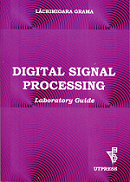## Digital Signal Processing - 4th year TTS

### Lecture

 Lecture Notes in DIGITAL SIGNAL PROCESSING - C. Rusu, L. Grama, RISOPRINT, Cluj-Napoca 2009 Lecture 1 Course Overview. Introduction to Digital Signal Processing Lecture 2 Discrete-Time Signals and Systems Lecture 3 Analysis of Discrete-Time Linear Time-Invariant Systems Lecture 4 Direct-Form Implementation of Discrete-Time Systems. LTIS Characterized by Difference Equations Lecture 5 z-Transform Lecture 6 z-Domain Analysis of LTIS. Fourier Series for Discrete-Time Periodic Signals Lecture 7 Fourier Transform for Discrete-Time Aperiodic Signals and Frequency Domain Characteristics of LTIS Lecture 8 Discrete Fourier Transform Lecture 9 Fast Fourier Transform Lecture 10 LTIS as Frequency Selective Filters. Linear-Phase FIR Filters Lecture 11 Design of Digital Filters Lecture 12 Direct Design Methods for IIR Systems. Implementation of Discrete-Time FIR Systems Lecture 13 Implementation of Discrete-Time IIR Systems Lecture 14 Digital Signal Processing Summary. Exam Example

### Laboratory

DIGITAL SIGNAL PROCESSING -- laboratory guide - L. Grama, U.T.PRESS, Cluj-Napoca 2014

Introduction to MATLABDiscrete-Time Signals

Sampling of Analog Signals. Correlation

Discrete-Time Linear Time-Invariant Systems

Fourier Transform and Discrete Fourier Transform

Laboratory 6
Test 1 - from laboratories 1, 2, 3, 4 and 5

Linear and Circular Convolution

Finite Impulse Response Filters. Design Methods

Discrete-Time Linear Time-Invariant Systems as Frequency Selective Filters

Infinite Impulse Response Filters. Indirect Design Methods

Infinite Impulse Response Filters. Direct Design Methods

Laboratory 12
Test 2 - from laboratories 7, 8, 9, 10 and 11

Laboratory 13
Seminar - Implementation of Discrete-Time Systems

Laboratory 14
Seminar

### References

 The complete list of References is available here

### Problems

 Solved problems Proposed problems

### Exams (january - february 2021)

 Questions: Exam: ReExam: ReReExam: